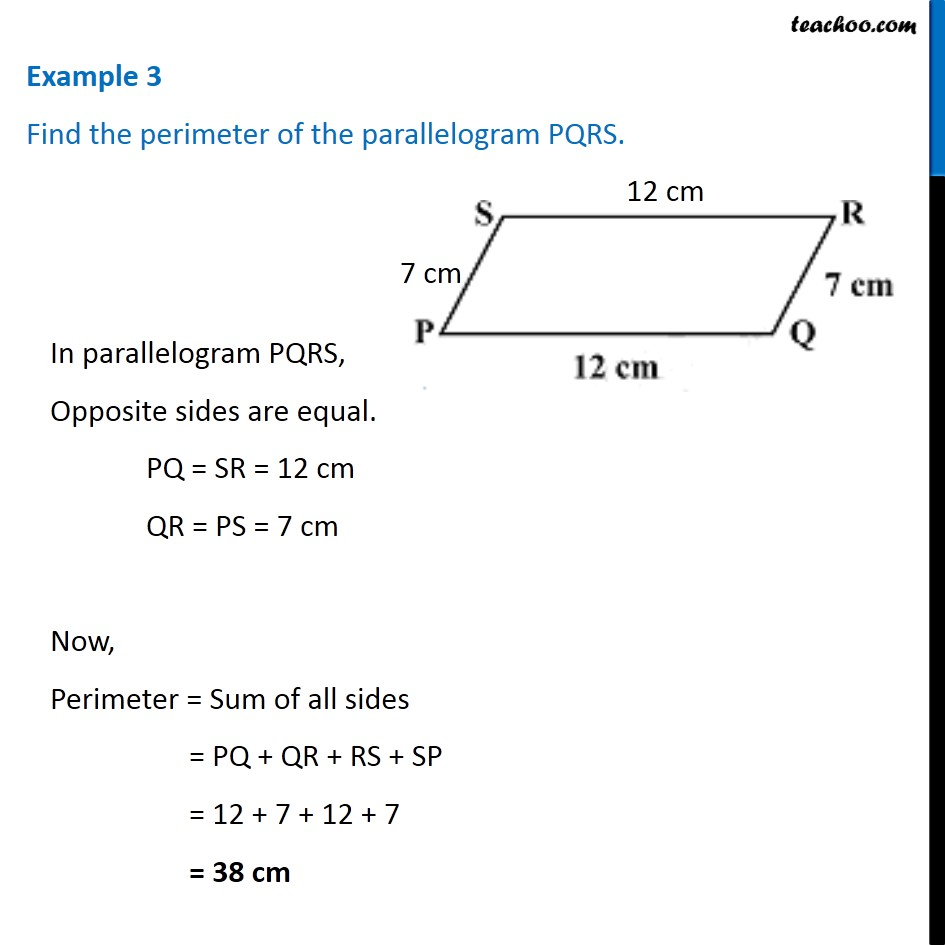1. Chapter 3 Class 8 Understanding Quadrilaterals
2. Concept wise
3. Parallelogram

Transcript

Example 3 Find the perimeter of the parallelogram PQRS. In parallelogram PQRS, Opposite sides are equal. PQ = SR = 12 cm QR = PS = 7 cm Now, Perimeter = Sum of all sides = PQ + QR + RS + SP = 12 + 7 + 12 + 7 = 38 cm

Parallelogram

About the AuthorDavneet Singh
Davneet Singh is a graduate from Indian Institute of Technology, Kanpur. He has been teaching from the past 9 years. He provides courses for Maths and Science at Teachoo.